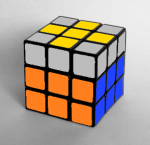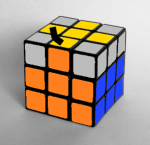# Solving the Top Layer Edges of the Rubik's CubeIn the fourth lesson on the Rubik's Cube (the penultimate solving lesson), progress is made on the top layer, continuing on from the previous lessons in which the first two layers were solved. This stage will solve the entirety of the cube except the last four corners. The top layer has many different solving methods, but the one chosen is similar to the first stage, the cross, so it will help students understand what they are doing better.

## Recap and Overview

As always, the lesson is begun with a recap of the previous lesson's content. Here the student is tested on some concepts and algorithms that they have learnt in each different lesson, and encourages them to explain the purpose of algorithms they provide. The answers are then provided on the following slide.

This lesson is very similar to the previous one, as two stages of the cube are solved together. Following the standard recap questions, the student is introduced to the two stages they will be working towards today. After this, a secondary recap is given to ensure that they can still solve the cube up to the starting point of this lesson. This is essential as trying to understand new concepts can be difficult when you're still stuck on a previous stage. Make sure the students can all still solve the cube up to this point otherwise they will not benefit as much from this lesson.

## Orienting and Permuting the Top Layer Edges

Both of these stages work on solving the top four edges without disturbing what has already been made. For the first stage, the edges are manipulated so that they all face the same direction. In the second stage, a single algorithm is use to swap two edges at a time to eventually solve all four.

Theorientation of the last layer edges stage provides both a one algorithm and a two algorithm method. This is done so that those who enjoy learning algorithms can use two to make a stage quicker to complete, whereas those who prefer repeating one algorithm to solve a certain stage have the option to do that also.

For each of the 3 different cases that require algorithms, an animation is provided that shows each choice (one algorithm/two algorithms). As these algorithms are very similar, it is suggested that a student tries to learn both to avoid unnecessary repetition (especially if they want to be able to solve the cube quickly).

The second stage involves thepermutation of the top layer edges. This stage is fairly simple as only one algorithm is used to swap two edges around. For the most part, the student will have to figure out which edges to swap by themselves, as there are many unique cases for this stage. However, the algorithm shouldn't be needed more than twice, as there aren't many different positions the edges can be in.

This stage is similar to the cross – They both have one algorithm, but the student needs to do most of the work, deciding when and where to use that algorithm. The algorithm is there to help the student, however they need to understand it and apply it correctly to be able to consistently solve this stage.

#### Rubik's Cube Curriculum SeriesDownload The PowerPoint Presentations from Google Drive